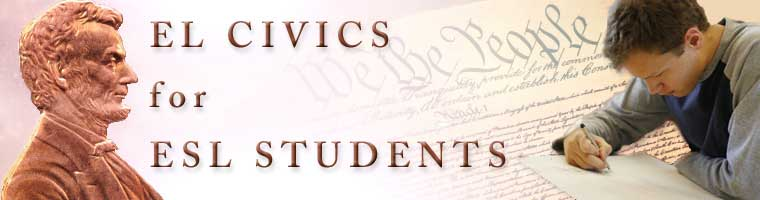# Count by Tens - Page 2

Thirty

thirty = 30

10 + 20 = 30

Ten plus twenty equal thirty.

Forty

forty = 40

10 + 30 = 40

Ten plus thirty equal forty.

Fifty

fifty = 50

10 + 40 = 50

Ten plus forty equal fifty.

## Lesson Activities

Numbers Crossword 1-50  Answer Key Count by Tens PowerPoint